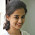## Search this site

### Video Lectures on "Pulse width Modulation for Power Electronic Converters" by Dr. G. Narayanan sir IISc Bangalore

Video Lecture Series from IIT Professors :
Pulse width Modulation for Power Electronic Converters by Dr. G. Narayanan sir

### Dr. G. Narayanan sir

Associate Professor
Room No: 234 First Floor
Power Electronics Group Department of Electrical Engineering Indian Institute of Science, Bangalore

 2000 Ph.D. Indian Institute of Science, Bangalore 1994 M.Tech. Indian Institute of Technology, Kharagpur 1992 B.E. College of Engineering, Guindy, Anna University, Chennai

 COURSE OUTLINE Converter topologies for AC/DC and DC/AC power conversion, overview of applications of voltage source converters; pulsewidth modulation techniques for 1-phase and 3-phase bridge converters, sine-triangle PWM, bus clamping PWM, space vector based PWM, advanced PWM techniques; calculation of switching and conduction losses; compensation for dead time and DC voltage regulation; estimation of current ripple and torque ripple in inverter fed drives; overmodulation; extension of modulation methods to multilevel inverters.

Detailed syllabus:

1. Power electronic converters for dc-ac and ac-dc power conversion (6 lectures).

Electronic switches, dc-dc buck and boost converters, H-bridge, multilevel converters – diode clamp, flying capacitor and cascaded-cell converters; voltage source and current source converters; evolution of topologies for dc-ac power conversion from dc-dc converters.
2. Applications of voltage source converters (2 lectures).

Overview of applications of voltage source converter, motor drives, active front-end converters, reactive compensators, active power filters
3. Purpose of pulsewidth modulation (2 lectures).

Review of Fourier series, fundamental and harmonic voltages; machine model for harmonic voltages; undesirable effects of harmonic voltages – line current distortion, increased losses, pulsating torque in motor drives; control of fundamental voltage; mitigation of harmonics and their adverse effects
4. Pulsewidth modulation (PWM) at low switching frequency (3 lectures).

Square wave operation of voltage source inverter, PWM with a few switching angles per quarter cycle, equal voltage contours, selective harmonic elimination, THD optimized PWM, off-line PWM
5. Triangle-comparison based PWM (4 lectures).

Average pole voltages, sinusoidal modulation, third harmonic injection, continuous PWM, bus-clamping or discontinuous PWM
6. Space vector based PWM (4 lectures).

Space vector concept and transformation, per-phase methods from a space vector perspective, space vector based modulation, conventional space vector PWM, bus-clamping PWM, advanced PWM, triangle-comparison approach versus space vector approach to PWM
7. Analysis of line current ripple (4 lectures).

Synchronously revolving reference frame; error between reference voltage and applied voltage, integral of voltage error; evaluation of line current ripple; hybrid PWM for reduced line current ripple
8. Analysis of dc link current (2 lectures).

Relation between line-side currents and dc link current; dc link current and inverter state; rms dc current ripple over a carrier cycle; rms current rating of dc capacitors
9. Analysis of torque ripple (2 lectures).

Evaluation of harmonic torques and rms torque ripple, hybrid PWM for reduced torque ripple
10. Inverter loss (3 lectures).

Simplifying assumptions in evaluation of inverter loss, dependence of inverter loss on line power factor, influence of PWM techniques on switching loss, design of PWM for low inverter loss.
11. Effect of inverter dead-time effect (2 lectures).

Requirement of dead-time, effect of dead-time on line voltages, dependence on power factor and modulation method, compensation of dead-time effect.
12. Overmodulation (3 lectures).

Per-phase and space vector approaches to overmodulation, average voltages in a synchronously revolving d-q reference frame, low-frequency harmonic distortion
13. PWM for multilevel inverter (3 lectures).

Extensions of sine-triangle PWM to multilevel inverters, voltage space vectors, space vector based PWM, analysis of line current ripple and torque ripple

Lecture schedule – Pulsewidth Modulation for Power Electronic Converters

 Overview of power electronic converters 1. Electronic switches. 2. DC - DC converters. 3. DC - AC voltage source inverter. 4. Voltage source and current source inverters. 5. Multilevel inverter - diode clamped inverter.- 1 6. Multilevel inverter - flying capacitor inverter - 2.  Multilevel inverter - flying capacitor inverter.- 3 Applications of voltage source converter 7. Applications of voltage source converter – I (motor drives, active rectifiers). 8. Applications of voltage source converter – II (reactive current and harmonic compensation).  Applications of voltage source converter – III Purpose of pulsewidth modulation (PWM) 9. Review of Fourier series. 10. Harmonic voltages and their undesirable effects. Pulsewidth modulation at low switching frequency 11. Low switching frequency operation of a VSI – I (square wave operation, one switching angle per quarter). 12. Low switching frequency operation of a VSI – II (Two switching angles per quarter). 13. Selective harmonic elimination and THD optimized PWM. Off-line optimized pulsewidth modulation Triangle-comparison based PWM 14. Sine-triangle modulation. 15. Third harmonic injection PWM (THIPWM). 16. Bus-clamping PWM - I. 17. Bus-clamping PWM - II. Space vector-based PWM 18. Concept of space vector. 19. Conventional space vector PWM and bus-clamping PWM. 20. A unified perspective of triangle-comparison and space vector based PWM. 21. Advanced bus-clamping PWM. Analysis of line current ripple 22. Transformation from stationary reference frame to synchronously revolving dq reference frame. 23. Volt-second balance and instantaneous error voltage. 24. Calculation of RMS line current ripple. 25. Space vector-based hybrid PWM for reduced line current ripple. Analysis of dc link current 26. Inverter state and dc link current. 27. Average and RMS values of dc link current. Analysis of torque ripple 28. Calculation of harmonic torques and RMS torque ripple. 29. Hybrid PWM techniques to reduce ripple torque. Inverter loss 30. Evaluation of conduction loss. 31. Dependence of switching loss on power factor and modulation method. 32. PWM techniques for reduced switching loss. Effect of inverter dead-time 33. Effect of dead-time with continuous modulation. 34. Effect of dead-time with discontinuous or bus-clamping PWM. Overmodulation 35. Per-phase approach to overmodulation. 36. Space vector approach to overmodulation. 37. A perspective from the synchronously revolving d-q reference frame. PWM for multilevel inverters 38. Extension of sine-triangle modulation to three-level inverters. 39. Extension of conventional space vector modulation to three-level inverters. 40. Analysis of line current ripple and torque ripple.

1.2.